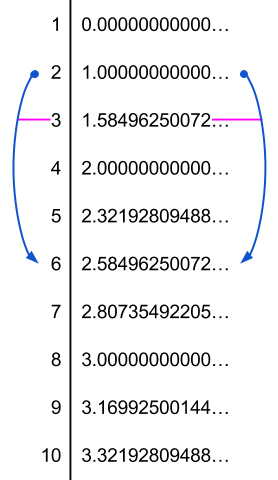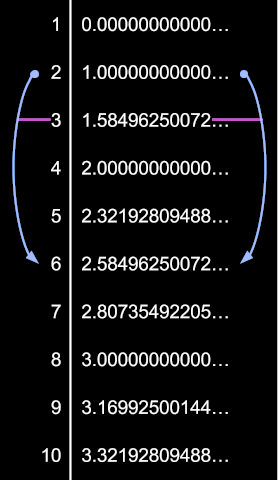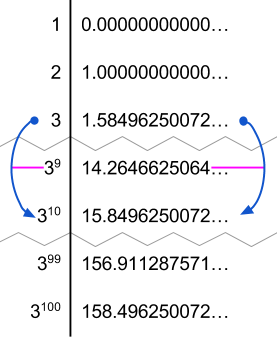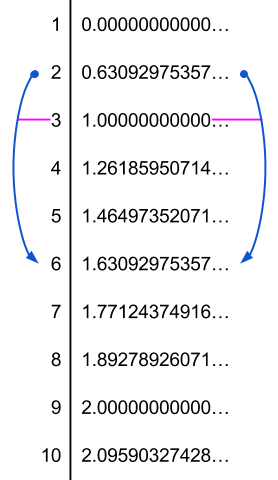# The log lattice

Log as the change in the cost of communicating and other pages give physical interpretations of what logarithms are really doing. Now it’s time to understand the raw numerics. Logarithm functions often output irrational, transcendental numbers. For example, $$\log_2(3)$$ starts with

1.5849625007211561814537389439478165087598144076924810604557526545410982277943585625222804749180882420909806624750591673437175524410609248221420839506216982994936575922385852344415825363027476853069780516875995544737266834624612364248850047581810676961316404807130823233281262445248670633898014837234235783662478390118977006466312634223363341821270106098049177472541357330110499026268818251703576994712157113638912494135752192998699040767081539505404488360

What do these long strings of digits mean? Why these numbers, in particular? We already have the tools to answer those questions, all that remains is to put them together into a visualization. In Fractional digits, we saw why it is that $$\log_2(3)$$ must be this number: The cost of a 3-digit in terms of 2-digits is more than 1 and less than 2; and the cost of ten 3-digits = one $$3^{10}$$-digit in terms of 2-digits is more than 15 and less than 16, and the cost of a hundred 3-digits = one $$3^{100}$$-digit in terms of 2-digits is more than 158 and less than 159, and so on. The giant number above is telling us about the entire sequence of $$3^{n}$$-digit costs, for every $$n$$. If we double it, we get the cost of a 9-digit in terms of 2-digits. If we triple it, we get the cost of a 27-digit in terms of 2-digits.

In fact, this number also interacts correctly with all the other outputs of $$\log_2.$$ $$\log_2(2)=1,$$ and if we add 1 to the number above, we get the cost of a 6-digit in terms of 2-digits.

That is, the outputs of the log base 2 form a gigantic lattice, with an often-irrational output corresponding to each input. The gigantic lattice is set up just so, such that whenever you start at $$x$$ on the left and go to $$x \cdot y,$$ the output you get on the right is the output to that corresponds to $$x$$ plus the output that corresponds to $$y$$.The values of the logarithm base 2 on integers from 1 to 10.

The log lattice is this giant lattice assigning a specific number to each number, such that multiplying on the left corresponds to adding on the right.An illustration of some of the connections between the logs base 2 of powers of 3.

It’s an intricate lattice that preservers a huge amount of structure — all the structure of the numbers, in fact, given that $$\log$$ is invertible.$$\log_2(3)$$ is a number that is simultaneously $$1$$ less than $$\log_2(6),$$ and half of $$\log_2(9)$$, and a tenth of $$\log_2(3^{10}),$$ and $$\log_2(3^9)$$ less than $$\log_2(3^{10}).$$ The value of $$\log_2(3)$$ has to satisfy a massive number of constraints, in order to be precisely the number such that multiplication on the left corresponds to addition on the right. It’s no surprise, then, that it has no concise decimal expansion.Notice the connections between the logs base 2 of the powers of 3. log 2 of 3 is a tenth of log 2 of 310 is a tenth of the log 2 of 3100, which is twice the log 2 of 350 which is twice the log 2 of 325. And, of course, the log 2 of 3101 is about 1.58 units larger than the log 2 of 3100.

In fact, the constraints on this log lattice are so tight that there’s only one way to do it, up to a multiplicative constant. You can multiply everything on the right side by a constant, and that’s the only thing you can do without disturbing the structure. For example, here’s part of the log lattice viewed in base 3:It’s exactly the same as the log lattice viewed in base 2, except that everything on the right has been divided by about 1.58 (i.e., multiplied by about 0.63).

What are logarithms doing? Well, fundamentally, there is a way to transform numbers such that what was once multiplication is now addition. If you were going to do multiplication to some numbers, you can transform them into this lattice, and then do addition, and then transform them back, and you’ll get the same result. There’s only one way to preserve all that structure, although if you want to view the structure, you need to (arbitrarily) choose some number on the left to call “1” on the right. Given that choice $$b$$ of translation, the log base $$b$$ taps into that intricate lattice.

Parents:

• Wait, really? Is this a joke or does being transcendental follow from having to satisfy many constraints?

• Huge “aha” here!